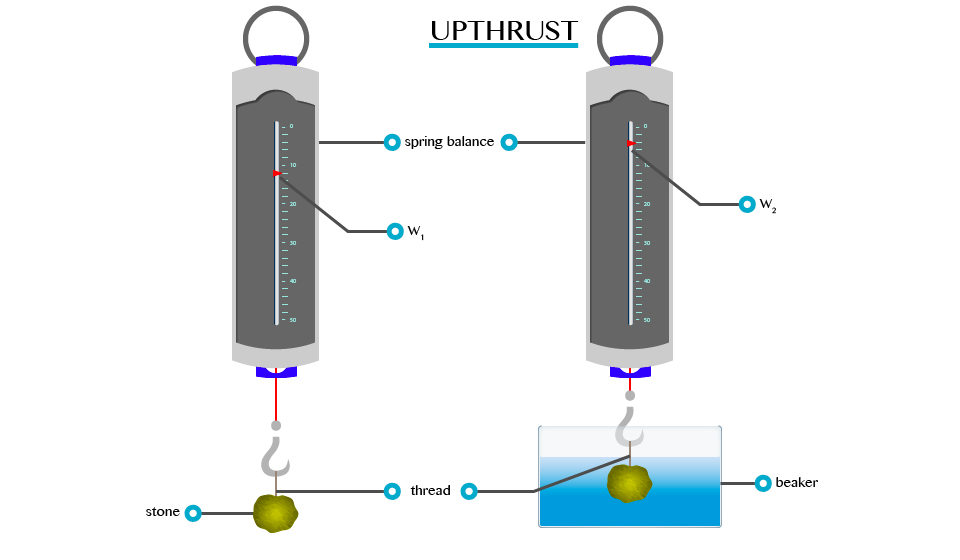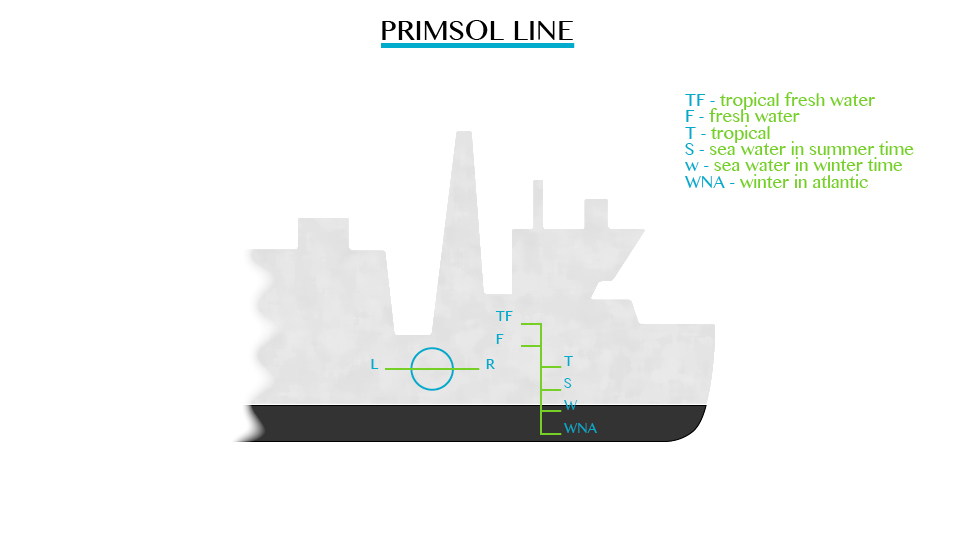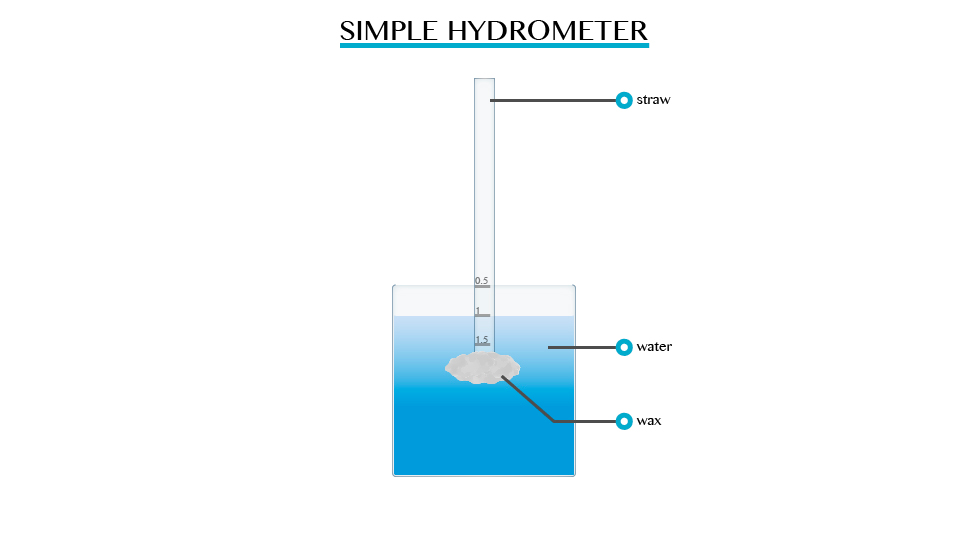Type Here to Get Search Results !

# PHYSICS FORM ONE TOPIC 5: ARCHIMEDES' PRINCIPLE AND LAW OF FLOTATIONARCHIMEDES' PRINCIPLE AND LAW OF FLOTATION
Archimedes' principle indicates that the upward buoyant force that is exerted on a body immersed in a fluid, whether fully or partially submerged, is equal to the weight of the fluid that the body displaces.
The Concept of Upthrust
Explain the concept of upthrust
If a heavy object is lifted while immersed in water, it may be raised more easily than when outside the water. This is due to the reason that when anything is placed in liquid, it receives an upward force called upthrust. A body appears to have lost some weight when immersed in water due to the upthrust exerted on the body by the water.
• By definition upthrust is referred as an upward force exerted by the body when it’s partially or totally immersed in water.
• Consider the experiment below to verity the concept of upthrust.
From this experiment, it will be observed that W1>W2. This is because:When a body is partially or totally immersed in any liquid, the liquid exerts an upward force.
A weight recorded on the spring balance of a body that is totally or partially immersed in any liquid is called apparent weight. E.g. W2 and the force, which temporally reduces the weight of the body, are called upthrust (u).
Verification of the Archimedes' Principle
Verify the archimedes principle
This is the principle which shows the relationship between the upthrust acting on a body and the weight of fluid it displaces when partially or completely immersed in the fluid. It was first discovered by a Greek scientist called Archimedes (287 to 121 BC).
• The principle states that, ”when a body is partially or totally immersed in a fluid it experiences an upthrust which is equal to the weight of the fluid displaced.”
• This principle can be verified by the following experiment.
Results:Weight of a body in air = W1Verification of the Archimedes' Principle
Weight of a body in water = W2 (apparent weight)
Uptrust = Loss of weight in water
U = W1 –W2
Example 1
Weight of a body in air = 10.0N
Weight of a body when immersed in water = 9.2N find the upthrust.
Solution:
Data given
Weight of a body in air (W1)= 10.0N
Weight of a body when in water (W2) = 9.2N
Formula
Upthrust = Loss of weight in water = W1-W2
= 10.0N – 9.2N
= 0.8N
The upthrust is 0.8
Example 2
The weight of a body when totally immersed in liquid is 4.2N. if the weight of the liquid displaced is 2.5N, find the weight of the body in the air.
Solution;
Data;
Apparent weight (W2) = 4.2N
Weight of liquid displaced (u) = 2.5N
Weight of body in air=?
Formular;
U = W1 –W2
W1= 4.2 +2.5 = 6.7N
Weight of body in air is 6.7N
The Archimedes' Principle in Determining Relative Density
Apply the archimedes principle to determine relative density
Relative density (R.D) of a substance can be defined as a ratio of the mass of a certain volume of the substance to the mass of an equal volume of water.
Relative density = Mass or weight of given volume of a substance overMass or weight of an equal volume of water.
R.D = weight of a substance in air over Weight of displaced water.
R.D = weight of a substance in air
From Archimedes’ principle the weight of an equal volume of water is equal to the weight of water displaced by the object, which is equal to the upthrust loss in weight. By weighing an object in air and then in water, the relative density can be determined.
Weight of a body in air = W1
Weight of a body in water = W2
Apparent loss in weight = W1-W2
Relative density = W1/W1 - W2
Example 3
A piece of glass weigh 1.2N in air and 0.7N when completely immersed water. Calculate its:
1. Relative density
2. Density of a glass
Given that density of water = 1000kg/cm3
And acceleration due to gravity = 10N/kg
Solution
Weight of the glass in air (W1) = 1.2N
Weight of the glass in water (W2) = 0.7N
R.D = W1/W1 – W2
1.2N/(1.2 – 0.7)
1.2/0.5
R.D = 2.4
R.D = Density of glass/Density of water
Density of a glass = R.D x Density of water
= 2.4 x 1000kg/cm
= 2400kg/m3
NB: Relative density has no SI unit
Difference between Floating and Sinking of Objects
Distinguish floating and sinking of objects
As we have discussed in upthrust, different objects with different density can sink or float. The object with higher density than water density will sink while that object with a density lower than water’s density will float. For example, a coin sinks in water and a large ship floats on water.
The Conditions for a Substance to Float in Fluids
Explain the conditions for a substance to float in fluids
When an object is completely or partially immersed in fluids, there are two forces acting on it, the weight (W) acting downwards and the upstrust (u) acting upwards. Refer to the figure below:
ConditionsConditions for a body to float include:
1. If W>U, there is downward movement of the body which is termed as sinking.
2. If W
3. If W=U, the body is equilibrium under the action of two equal and opposite force. Thus, the body floats.
Relationship between Upthrust and Weight of Floating Body
Relate upthrust and weight of floating body
The relationship can be determined by considering the following experiment.
Upthrust and Weight of Floating BodyPrinciple of Floatation
State the law of flotation
The above experiment shows that, the upthrust is equal to the weight of the liquid displaced and therefore the upthrust is equal to the weight of the floating body as the two forces are equal.
The mass of the floating body is equal to the mass of fluid displaced; in the above experiment is the same as the weight of the piece of wood. This result is agreement with the principle of floatation.
The principle of floatation states “A floating body displaces a weight of the fluid which is equal to its own weight.”
The Law of Floatation in Everyday Life
Apply the law of flotation in everyday life
Floating of ship
Iron is much denser than water and a block of iron sinks immediately in water. Ships are made with hollowness such that their total densities are less than that of water. Therefore, a ship displaces water equal to its weights.
The upthrust of the water is sufficient to support the weight of the ship. When the ship is loaded with cargo it sinks lower in the water. The volume of water displaced by the ship and its cargo depends upon whether it is floating in fresh water or in seawater. It floats lower in fresh water (R.D= 1.0) than in seawater (R.D=1.025) the mass of fresh water displaced.
For example, if a ship weighs 20,000 tons, then it must displace 20,000 tones of water to float. If 2,000 tones of cargo is added, the ship lowers in water until an extra 1,000 tons of water have been displaced
Plimsoll line;This line indicates the safe limit of loading. Many plimsoll lines may be marked on a ship to show minimum heights above different types of water in different seasons.Balloons
Figure below shows the type of balloons used to carry instruments to a high altitude for recording meteorological measurements when filled with gas. E.g. helium, it displaces a volume of air equal to its volume.
Air has greater density compared to the density of a gas in the balloon. Therefore, the weight of air displaced is greater than that of balloon. The balloon drifts up by a force, which is equal to the difference between the upthrust and the total weight of the balloon (W).
Submarine
The buoyancy of a submarine depends on the quantity of water in its ballast tanks. When it is required to drive, water is admitted to special tanks. When the water is ejected from the tanks by means of compressed air, the submarine raises to the surface and floats just like any other ship.
The mode of Action of a Hydrometer
Describe the mode of action of a Hydrometer
A hydrometer is an instrument used for measuring the densities of liquids such as milk, alcohol and acids. The greater the density of the liquid the shorter the stem of hydrometer immersed. Hydrometer sinks lower in kerosene and floats higher in water.
Construction of a Simple Hydrometer
Construct a simple Hydrometer
Single hydrometer can be made using pieces of straws or test tubes weighed down with wax.Hydrometer in Determining the Relative Density of Different Liquids
Use Hydrometer to determine the relative density of different liquids
The relative density of liquid is measured directly by using a suitable hydrometer, it contains:
1. A heavy sinker, containing mercury or lead shots that keep the hydrometer upright when it floats.
2. An air bulb to increase the volume of displaced liquid, and overcomes the weight of the sinker.
3. The stem is thin so that small changes in density give large differences in readings.
4. The hydrometer is made up of glass so it does not soak up liquids.

Tags